## 1任务介绍

• 监督算法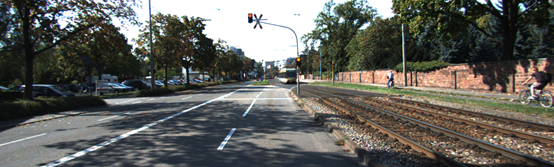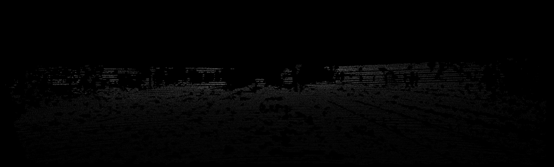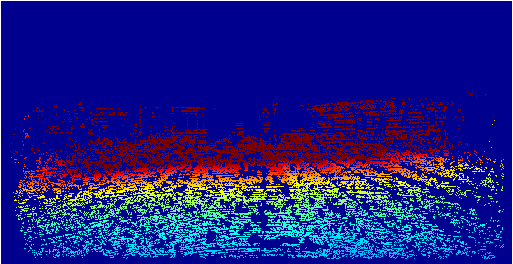• 无监督算法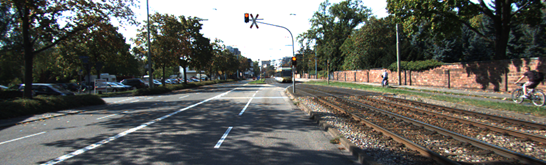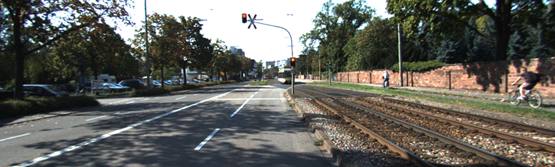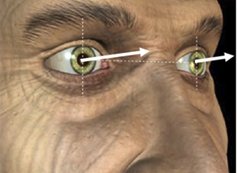• Structure from motion/基于视频的深度估计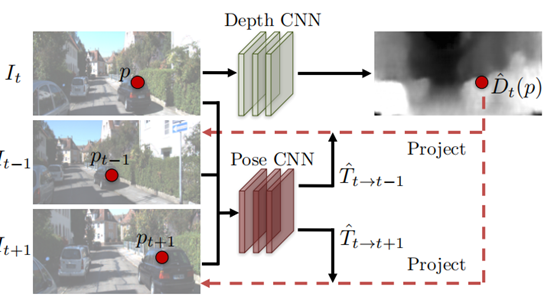## 2 数据集介绍

### 2.1 KITTI

KITTI是一个多任务属性的数据集，其中原始数据采集平台装配有2个灰度摄像机，2个彩色摄像机，一个Velodyne 64线3D激光雷达，4个光学镜头，以及1个GPS导航系统。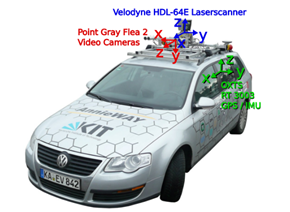### 2.2vKITTI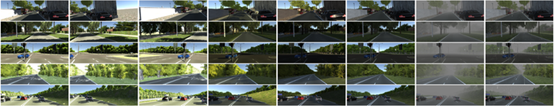### 2.3Cityscapes

Cityscapes的数据取自德国的50多个城市的户外场景，其中数据包含有左右视角图像、视差深度图、相机校准、车辆测距、行人标定、目标分割等，同时也包含有类似于vKITTI的虚拟渲染场景图像。其中简单的左视角图像、相机标定、目标分割等数据需要利用学生账号注册获取，其他数据需要联系管理员获取。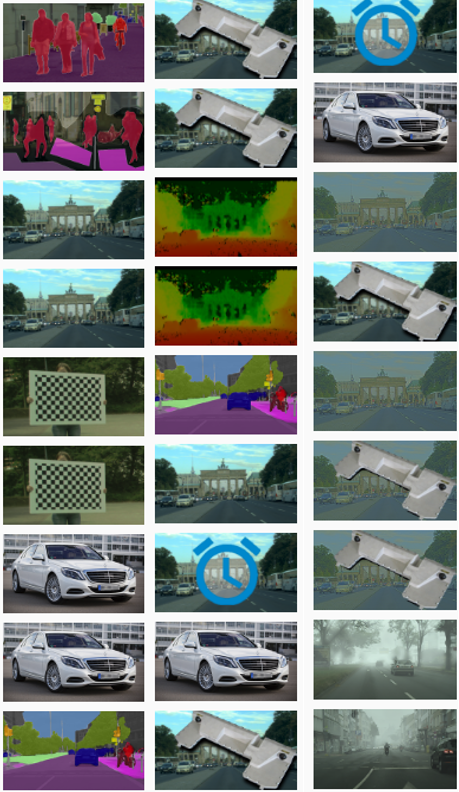### 2.4NYU Depth V2

NYU Depth V2数据集中包含有428G室内场景数据，同时包含有目标分割标注、深度标注。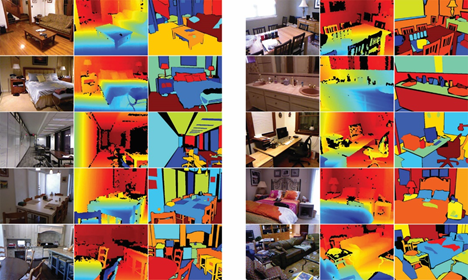### 2.5 ScanNet

ScanNet中包含有约1500个视频序列的RGB-D数据，主要用于三维重建。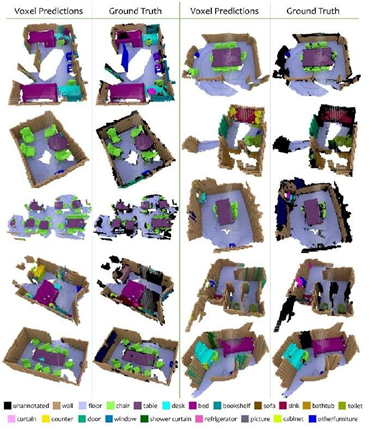### 2.6Make3D

Make3d数据集包含约1000张室外场景图片，50张室内场景，7000张合成物体。其中包含有激光-2D图像，立体图像、深度数据等。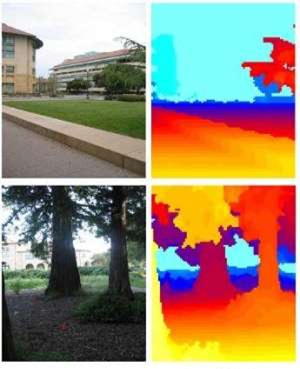## 3 数据处理

### 3.1数据组成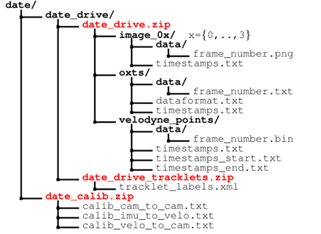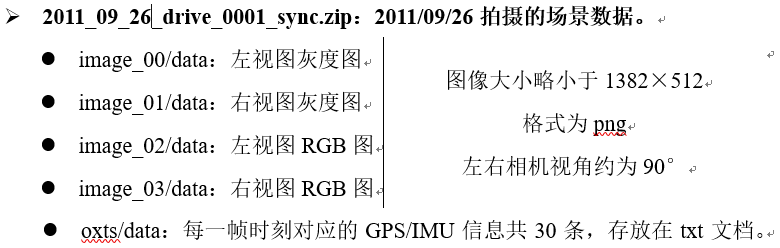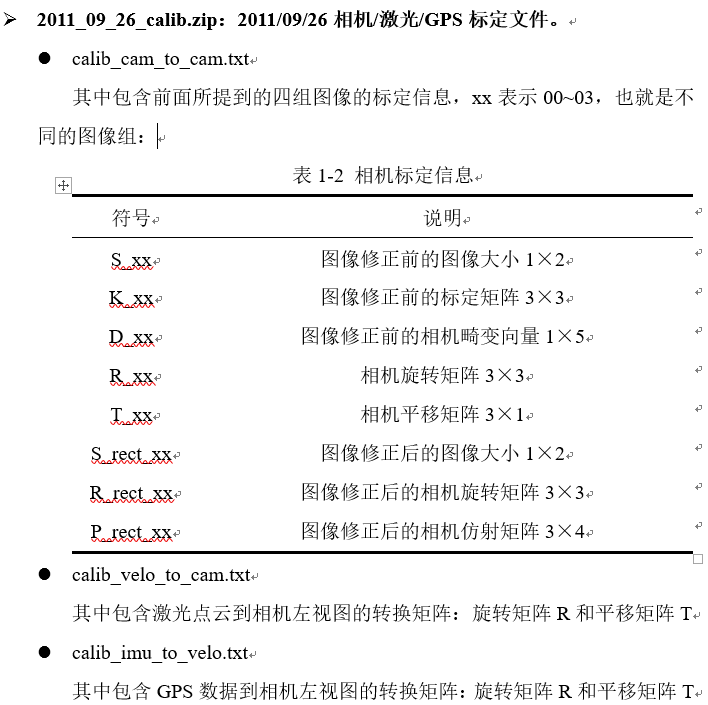### 3.2 数据处理

$$\begin{array}{l} imu2cam = \left[ {\begin{array}{*{20}{c}} {R\_rect\_00}&0\\ 0&1 \end{array}} \right] \times {\left[ {\begin{array}{*{20}{c}} R&T\\ 0&1 \end{array}} \right]_{velo2cam}} \times {\left[ {\begin{array}{*{20}{c}} R&T\\ 0&1 \end{array}} \right]_{imu2velo}}\\ scale = \cos \left( {latitude} \right)\\ t = \left[ {\begin{array}{*{20}{c}} {{t_x}}\\ {{t_y}}\\ {{t_z}} \end{array}} \right] = \left[ {\begin{array}{*{20}{c}} {scale \times longtitude \times \pi /180}\\ {scale \times er \times \log \left[ {\tan \left( {\frac{{90 + latitude}}{{360}}\pi } \right)} \right]}\\ {altitude} \end{array}} \right]\\ roll,yaw,pitch \Rightarrow R = {R_z}{R_y}{R_x}\\ \Rightarrow T = \left[ {\begin{array}{*{20}{c}} R&t\\ 0&1 \end{array}} \right]\\ \Rightarrow {T_{t \to s}} = imu2ca{m_t} \times T_s^{ - 1} \times {T_t} \times imu2cam_t^{ - 1} \end{array}$$

$$\begin{array}{l} \left\{ \begin{array}{l} P\_rect\_00[0,:] * = zoom\_x\\ P\_rect\_00[1,:] * = zoom\_ \end{array} \right.y\\ \Rightarrow K = P\_rect\_00[:,:3] \end{array}$$

$$P\_velo2im = P\_rect\_00 \times R\_rect\_00 \times {\left[ {\begin{array}{*{20}{c}} R&T\\ 0&1 \end{array}} \right]_{velo2cam}}$$

$$\begin{array}{l} Z\left[ {\begin{array}{*{20}{c}} x\\ y\\ 1 \end{array}} \right] = {\left( {P\_velo2im \times \left[ {\begin{array}{*{20}{c}} {{X_v}}\\ {{Y_v}}\\ {{Z_v}}\\ 1 \end{array}} \right]} \right)^T} = \left[ {\begin{array}{*{20}{c}} {X'}\\ {Y'}\\ Z \end{array}} \right]\\ \Rightarrow x = X'/Z,y = Y'/ZZ = Z \end{array}$$

• 随机水平翻转，所以需要改变相机内参的水平平移量cx=w-cx；

• 随机尺度变换并剪切至固定大小：
$$\left[ {\begin{array}{*{20}{c}} {{f_x}}&{{c_x}}\\ {{f_y}}&{{c_y}} \end{array}} \right] = \left[ {\begin{array}{*{20}{c}} {{f_x} \times scal{e_x}}&{{c_x} \times scal{e_x} + offse{t_x}}\\ {{f_y} \times scal{e_y}}&{{c_y} \times scal{e_y} + offse{t_y}} \end{array}} \right]$$

### 3.3评价指标

KITTI数据集在考虑深度估计信息误差时，所以判定的时候只取0.40810811H ~ 0.99189189H，0.03594771W ~ 0.9640522229W部分图像区域，当然也经常会只取50m或者80m范围内的深度信息。为了让预测深度和真实深度的数量级范围一致，一般会用二者深度的中位数作为尺度，对预测深度信息进行尺度放缩。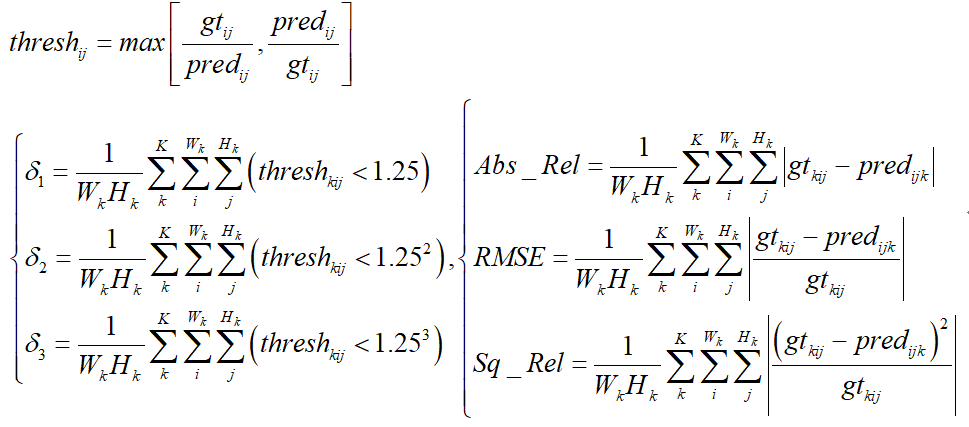## 4 相关工作

### 4.1基于单目视觉的深度估计

• 传统编解码结构

深度估计任务是从二维图像到二维深度图像的预测，因此整个过程是一个自编码过程，包含编码和解码，通俗点就是下采样和上采样。这类结构主要有FCN框架和U-net框架，二者的下采样过程都是利用了卷积和池化，而上采样利用了逆卷积/转置卷积(Deconvolution)和upsample。

• 深度回归网络

早期的单目深度估计网络框架基本上都是直接利用了上面所提到的两个基础框架进行了预测，为了让这类框架更好的应用于深度估计问题，一般都从以下几个方面着手：更好的backbone模型、多尺度、更深的网络。

以3DV 2016中《Deeper Depth Prediction with Fully Convolutional Residual Networks》一文为例，其提出了FCRN网络框架：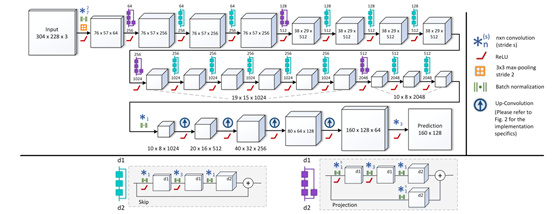其网络框架主体是基于Resnet-50，在上采样过程中采用了独特的方式，将逆卷积用up-pooing+conv的方式替代了，将Resnet中的project模块进行了一定的改进。

其中上采样过程中将特征图利用0元素进行填充，然后利用一类特殊的卷积核进行特征提取，这一过程可以先卷积，然后错位相连得到，原理如下：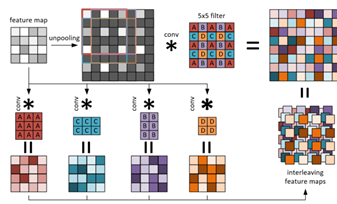其中可以发现卷积核并非是正方形，而是矩形，不过过程其实是一样的。而projection部分，即resnet中原图先经过1×1卷积再与特征图相连的部分，变为：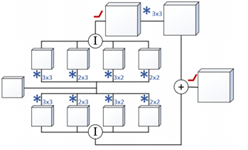具体细节我就不在多讲了，其效果如下：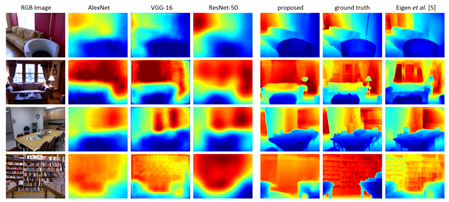• 深度分类网络

将深度估计问题变为回归问题的缺点在于，太依赖于数据集的场景，并且由于图像中深度往往是分层的，类似于等高线之类的，所以也有学者将深度估计变为一个分类问题，而类别数就是将最远实际距离划分为多份而制作的。

以此为代表的是CVPR2018中《Deep Ordinal Regression Network for Monocular Depth Estimation》所提出的DORN框架：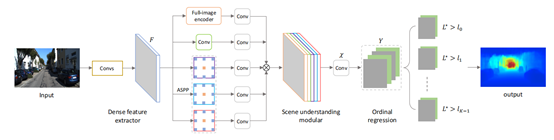该框架2018年在多个数据集上取得了第一的名次，不过现在有个别算法超越了。可以看到，原图在经过密集特征提取之后，增加了一个场景理解模块，这一模块包含5个部分。其中full-image encoder部分与传统的自编码器不同：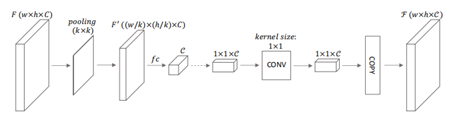可以看到其先是利用池化进行下采样，将其拉伸为向量之后，利用全连接层进行编解码，然后还原为原图大小。而ASPP模块是利用了膨胀卷积（dilated convolution）进行特征提取，膨胀倍数分别为6,12,18。五个部分concat得到最终的特征图。再进入有序回归模块，实质上是多分类器。其将深度范围划分为多个区间：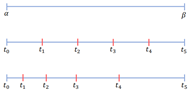最后输出W×H×2K的结果，K代表深度区间，2K是因为每两个相邻的通道值2n表示深度小于n的概率，2n+1表示深度大于n的概率，二者利用softmax归一化。

其效果如下：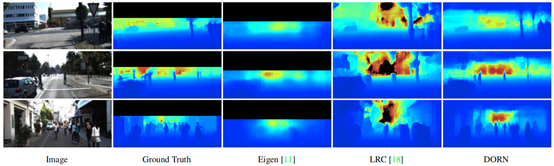可以看到，DORN对于深度的细节把握得非常好，其速度约为2fps，代码基于caffe平台，链接为：https://github.com/hufu6371/DORN

无论是回归还是分类，都是以深度信息作为标签的监督算法，因此其受限于训练集场景，仅限于刷榜。

### 4.2结合双目视觉的单目深度估计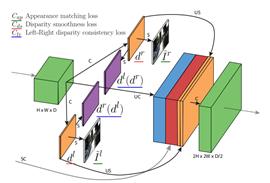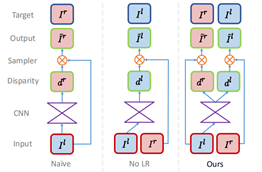• 外观匹配损失，即预测的视图和实际视图的区别：
$$C_{ap}^l = \frac{1}{N}\sum\limits_{i,j} {\alpha \frac{{1 - SSIM\left( {I_{ij}^l,\tilde I_{ij}^l} \right)}}{2}} + \left( {1 - \alpha } \right)\left\| {I_{ij}^l - \tilde I_{ij}^l} \right\|$$
其中SSIM指的是结构相似性。

• 视差平滑性约束：
$$C_{ds}^l = \frac{1}{N}\sum\limits_{i,j} {\left| {{\partial _x}d_{ij}^l} \right|} {e^{ - \left\| {{\partial _x}I_{ij}^l} \right\|}} + \left| {{\partial _y}d_{ij}^l} \right|{e^{ - \left\| {{\partial _y}I_{ij}^l} \right\|}}$$

• 左右视差一致性损失：
$$C_{lr}^l = \frac{1}{N}\sum\limits_{i,j} {\left| {d_{ij}^l - d_{ij + d_{ij}^l}^r} \right|}$$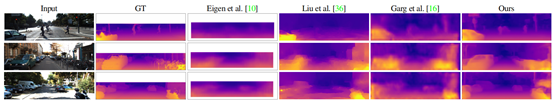SVS网络框架如下：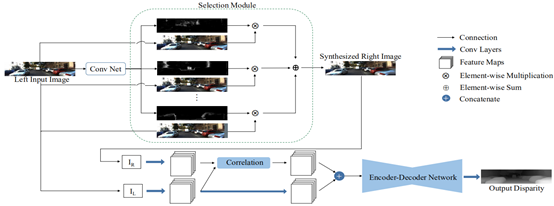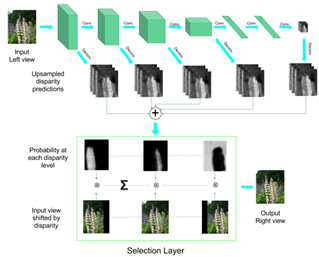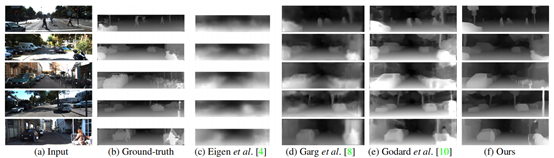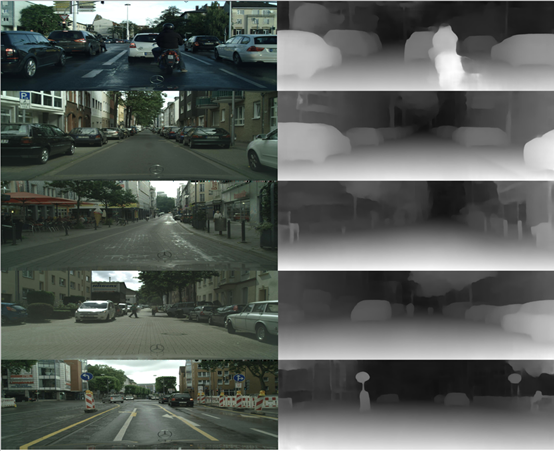### 4.3基于视频的相机位姿估计和视觉测距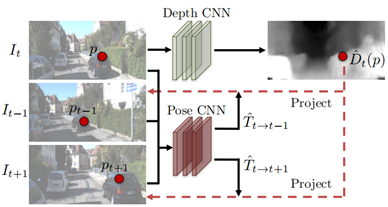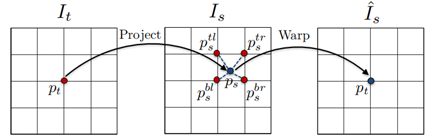$$\left\{ \begin{array}{l} {p_s} \sim K{{\mathord{\buildrel{\lower3pt\hbox{\scriptscriptstyle\frown}} \over T} }_{t \to s}}{{\mathord{\buildrel{\lower3pt\hbox{\scriptscriptstyle\frown}} \over D} }_t}\left( {{p_t}} \right){K^{ - 1}}{p_t}\\ {{\mathord{\buildrel{\lower3pt\hbox{\scriptscriptstyle\frown}} \over I} }_s}\left( {{p_t}} \right) = {I_s}\left( {{p_s}} \right) = \sum\nolimits_{i \in \left\{ {t,b} \right\},j \in \left\{ {l,r} \right\}} {{w^{ij}}{I_s}\left( {p_s^{ij}} \right)} \\ \sum\nolimits_{i,j} {{w^{ij}}} = 1 \end{array} \right.$$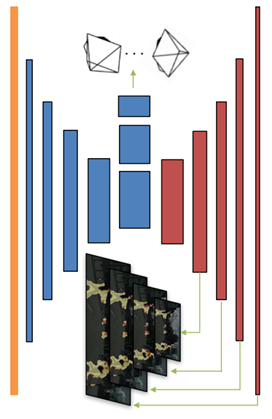$$\begin{array}{l} {L_{vs}} = \sum\limits_{\left\langle {{I_1},...,{I_N}} \right\rangle \in S} {\sum\limits_p {{{\mathord{\buildrel{\lower3pt\hbox{\scriptscriptstyle\frown}} \over E} }_s}\left( p \right)\left| {{I_t}\left( p \right) - {{\mathord{\buildrel{\lower3pt\hbox{\scriptscriptstyle\frown}} \over I} }_s}\left( p \right)} \right|} } \\ L = \sum\limits_l {L_{vs}^l} + {\lambda _s}L_{smooth}^l + {\lambda _e}\sum\limits_s {{L_{reg}}\left( {\mathord{\buildrel{\lower3pt\hbox{\scriptscriptstyle\frown}} \over E} _s^l} \right)} \end{array}$$
l指的是尺度，s指的是图片，其中的平滑性约束跟上一节所讲的Monodepth一样，由于解释性掩膜无标签，如果不加约束的话会自动为0，所以利用交叉熵损失函数对其进行了约束，默认为全1矩阵。其效果如下：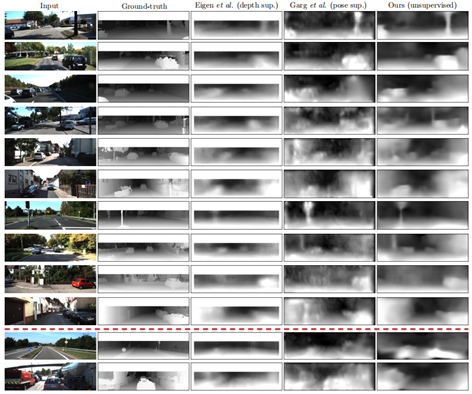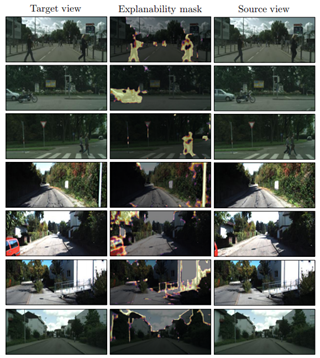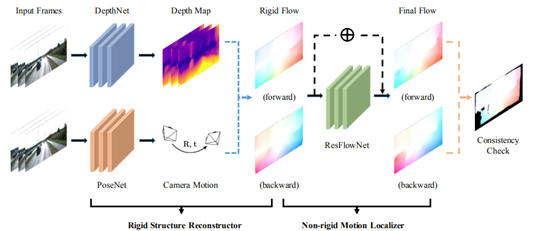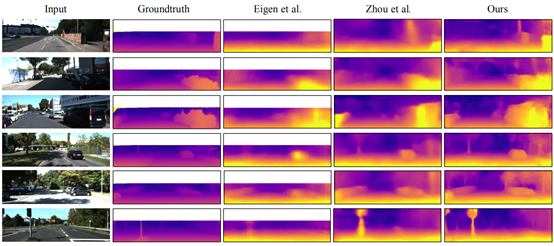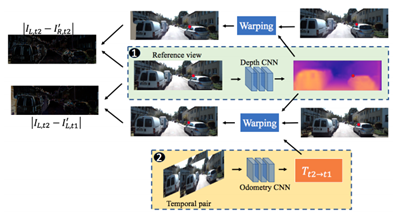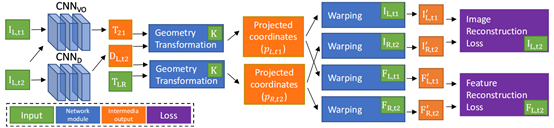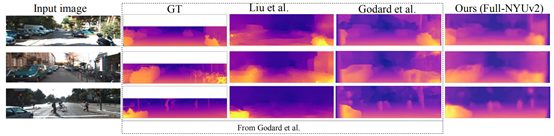### 4.4基于图像风格迁移的单目深度估计

ECCV2018中《T2Net: Synthetic-to-Realistic Translation for Solving Single-Image Depth Estimation Tasks》所提出的T2Net尝试性地将图像风格迁移引入单目深度估计领域，虽然效果只是2016年的水平，不过也算是一次很好的尝试了。下面介绍下T2Net的思路，首先给出其网络框架：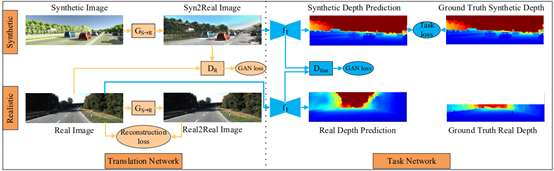• 由合成图像风格迁移生成的图像与原始图像的GAN Loss，即利用判别器进行判定的误差；
• 由真实图像风格迁移生成的图像预原始图像的重构误差，这一部分计算L1 Loss；
• 由合成图像风格迁移生成的图像与原始图像的编码特征的GAN Loss。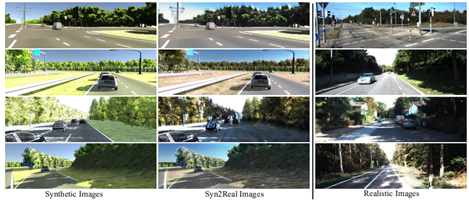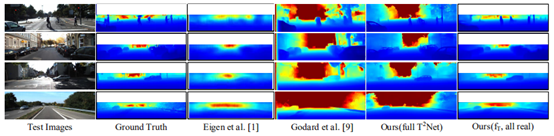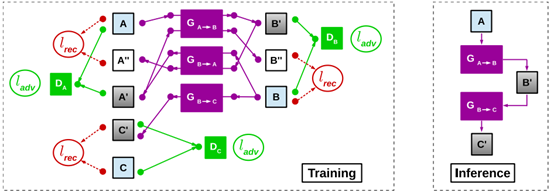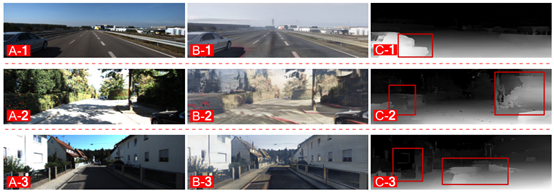### 4.5多任务深度估计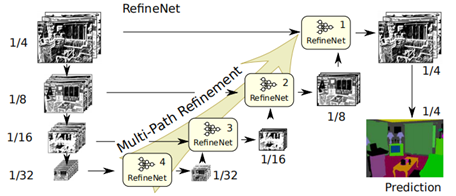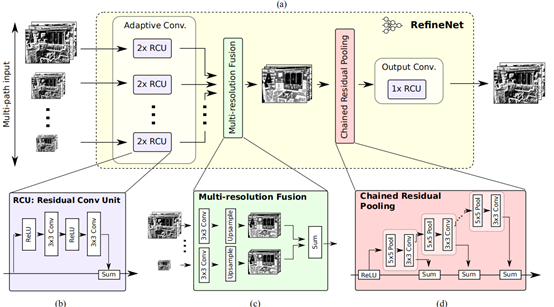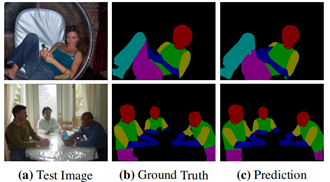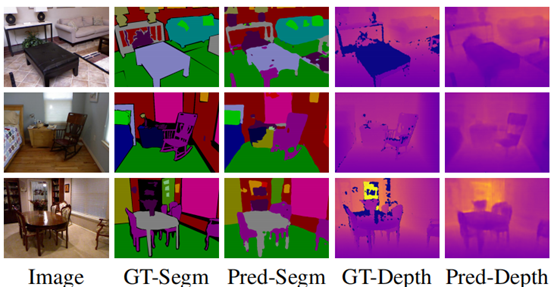ECCV2018中《DF-Net: Unsupervised Joint Learning of Depth and Flow using Cross-Task Consistency》一文提出了单目深度估计和光流预测的联合任务框架。不同于单独训练两个任务的方式，作者将二者的一致性进行了考虑，从而做到二者的相互促进，可以看到对比效果：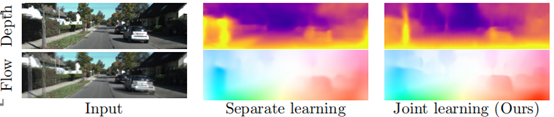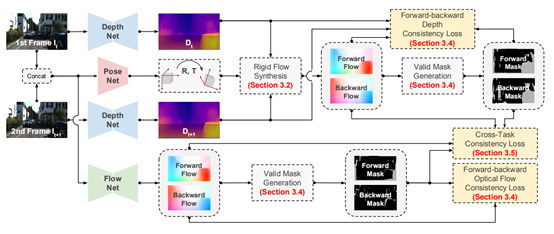​ 对于刚性流场景下的合成光流信息和直接预测到的光流信息，二者都反映了相邻两帧的像素点的对应关系，因此作者对此引入了光照约束（利用对比映射和插值，计算每个像素点的像素值差异）和深度的平滑性约束。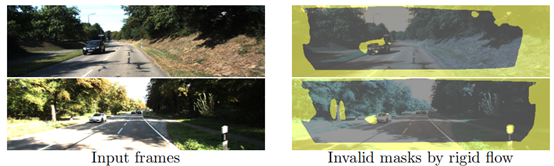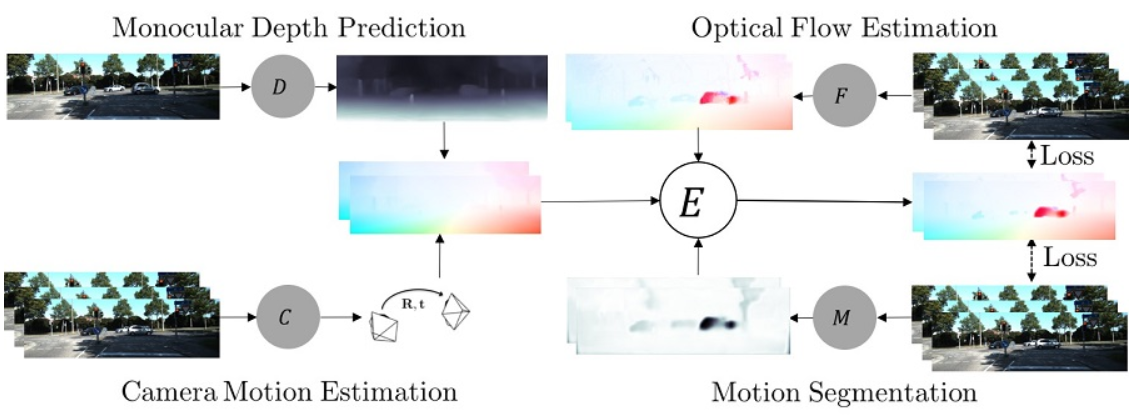## 5总结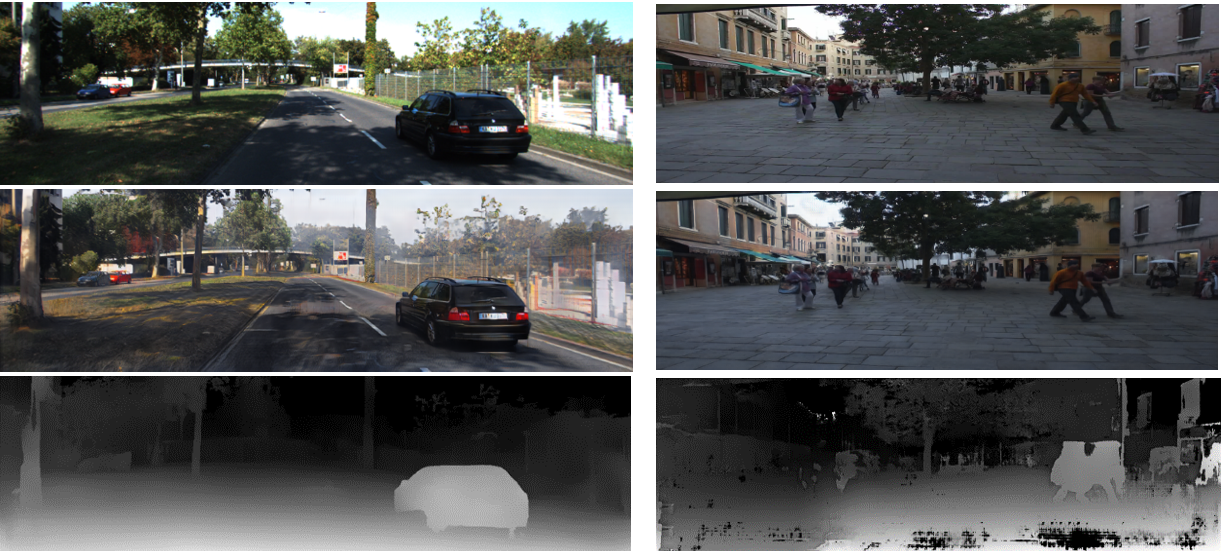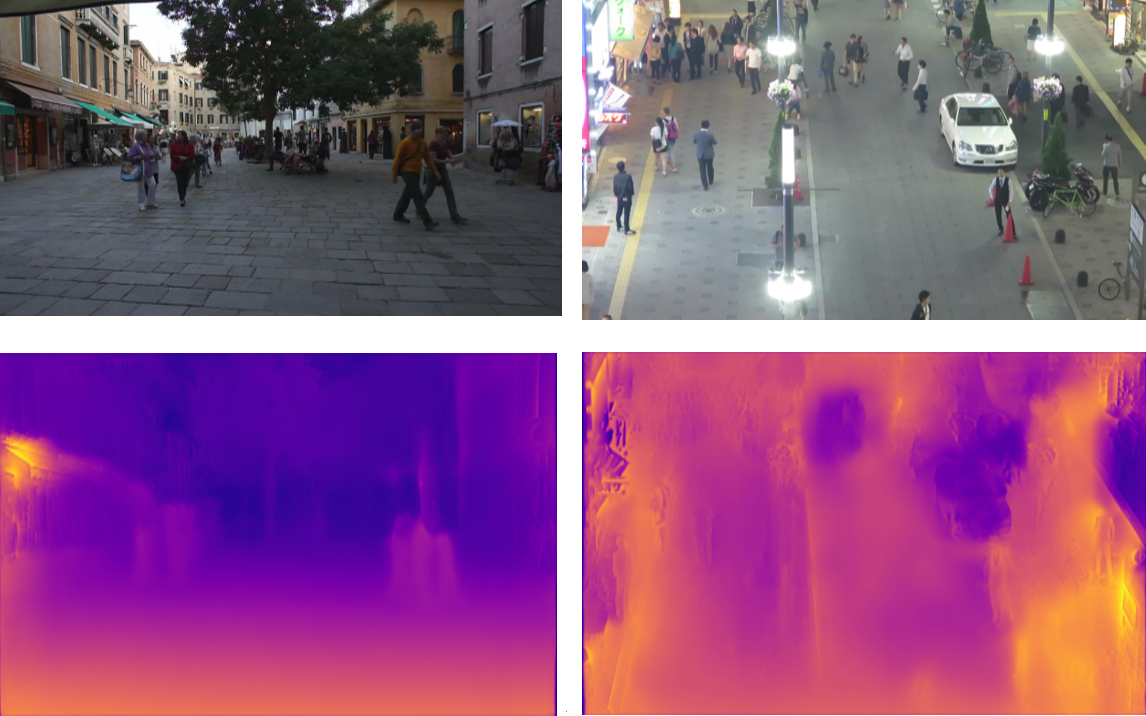## 6参考文献

 Long J, Shelhamer E, Darrell T. Fully convolutional networks for semantic segmentation[C]//Proceedings of the IEEE conference on computer vision and pattern recognition. 2015: 3431-3440.

 Ronneberger O, Fischer P, Brox T. U-net: Convolutional networks for biomedical image segmentation[C]//International Conference on Medical image computing and computer-assisted intervention. Springer, Cham, 2015: 234-241.

 Laina I, Rupprecht C, Belagiannis V, et al. Deeper depth prediction with fully convolutional residual networks[C]//2016 Fourth international conference on 3D vision (3DV). IEEE, 2016: 239-248.

 Fu H, Gong M, Wang C, et al. Deep ordinal regression network for monocular depth estimation[C]//Proceedings of the IEEE Conference on Computer Vision and Pattern Recognition. 2018: 2002-2011.

 Godard C, Mac Aodha O, Brostow G J. Unsupervised monocular depth estimation with left-right consistency[C]//Proceedings of the IEEE Conference on Computer Vision and Pattern Recognition. 2017: 270-279.

 Dosovitskiy A, Fischer P, Ilg E, et al. Flownet: Learning optical flow with convolutional networks[C]//Proceedings of the IEEE international conference on computer vision. 2015: 2758-2766.

 Ilg E, Mayer N, Saikia T, et al. Flownet 2.0: Evolution of optical flow estimation with deep networks[C]//Proceedings of the IEEE conference on computer vision and pattern recognition. 2017: 2462-2470.

 Mayer N, Ilg E, Hausser P, et al. A large dataset to train convolutional networks for disparity, optical flow, and scene flow estimation[C]//Proceedings of the IEEE Conference on Computer Vision and Pattern Recognition. 2016: 4040-4048.

 Xie J, Girshick R, Farhadi A. Deep3d: Fully automatic 2d-to-3d video conversion with deep convolutional neural networks[C]//European Conference on Computer Vision. Springer, Cham, 2016: 842-857.

 Luo Y, Ren J, Lin M, et al. Single View Stereo Matching[C]//Proceedings of the IEEE Conference on Computer Vision and Pattern Recognition. 2018.

 Zhou T, Brown M, Snavely N, et al. Unsupervised learning of depth and ego-motion from video[C]//Proceedings of the IEEE Conference on Computer Vision and Pattern Recognition. 2017: 1851-1858.

 Yin Z, Shi J. Geonet: Unsupervised learning of dense depth, optical flow and camera pose[C]//Proceedings of the IEEE Conference on Computer Vision and Pattern Recognition. 2018: 1983-1992.

 Zhan H, Garg R, Saroj Weerasekera C, et al. Unsupervised learning of monocular depth estimation and visual odometry with deep feature reconstruction[C]// Proceedings of the IEEE Conference on Computer Vision and Pattern Recognition. 2018: 340-349.

 Goodfellow I, Pouget-Abadie J, Mirza M, et al. Generative adversarial nets[C]//Advances in neural information processing systems. 2014: 2672-2680.

 Radford A , Metz L , Chintala S . Unsupervised Representation Learning with Deep Convolutional Generative Adversarial Networks[J]. Computer Science, 2015.

 Arjovsky M, Chintala S, Bottou L. Wasserstein gan[J]. arXiv preprint arXiv:1701.07875, 2017.

 Gulrajani I, Ahmed F, Arjovsky M, et al. Improved training of wasserstein gans[C]//Advances in Neural Information Processing Systems. 2017: 5767-5777.

 Mao X, Li Q, Xie H, et al. Least squares generative adversarial networks[C]//Proceedings of the IEEE International Conference on Computer Vision. 2017: 2794-2802.

 Mirza M, Osindero S. Conditional generative adversarial nets[J]. arXiv preprint arXiv:1411.1784, 2014.

 Isola P, Zhu J Y, Zhou T, et al. Image-to-image translation with conditional adversarial networks[C]//Proceedings of the IEEE conference on computer vision and pattern recognition. 2017: 1125-1134.

 Wang T C, Liu M Y, Zhu J Y, et al. High-resolution image synthesis and semantic manipulation with conditional gans[C]//Proceedings of the IEEE Conference on Computer Vision and Pattern Recognition. 2018: 8798-8807.

 Zhu J Y, Park T, Isola P, et al. Unpaired image-to-image translation using cycle-consistent adversarial networks[C]//Proceedings of the IEEE international conference on computer vision. 2017: 2223-2232.

 Wang T C , Liu M Y , Zhu J Y , et al. Video-to-Video Synthesis[J]. arXiv preprint arXiv:1808.06601,2018.

 Zheng C, Cham T J, Cai J. T2net: Synthetic-to-realistic translation for solving single-image depth estimation tasks[C]//Proceedings of the European Conference on Computer Vision (ECCV). 2018: 767-783.

 Atapour-Abarghouei A, Breckon T P. Real-time monocular depth estimation using synthetic data with domain adaptation via image style transfer[C]//Proceedings of the IEEE Conference on Computer Vision and Pattern Recognition. 2018: 2800-2810.

 Nekrasov V , Dharmasiri T , Spek A , et al. Real-Time Joint Semantic Segmentation and Depth Estimation Using Asymmetric Annotations[J]. arXiv preprint arXiv:1809.04766,2018.

 Nekrasov V , Shen C , Reid I . Light-Weight RefineNet for Real-Time Semantic Segmentation[J]. arXiv preprint arXiv:1810.03272, 2018.

 Lin G , Milan A , Shen C , et al. RefineNet: Multi-Path Refinement Networks for High-Resolution Semantic Segmentation[C]//Proceedings of the IEEE Conference on Computer Vision and Pattern Recognition.,2017:1925-1934

 Zou Y , Luo Z , Huang J B . DF-Net: Unsupervised Joint Learning of Depth and Flow using Cross-Task Consistency[C]//Proceedings of the European Conference on Computer Vision (ECCV). 2018:36-53.

 Ranjan A, Jampani V, Balles L, et al. Competitive collaboration: Joint unsupervised learning of depth, camera motion, optical flow and motion segmentation[C]//Proceedings of the IEEE Conference on Computer Vision and Pattern Recognition. 2019: 12240-12249.

-------------本文结束感谢您的阅读-------------